﻿ 船舶路径规划数值仿真 Numerical Simulation of Path Planning in Ship

Dynamical Systems and Control
Vol. 08  No. 02 ( 2019 ), Article ID: 29134 , 8 pages
10.12677/DSC.2019.82007

Numerical Simulation of Path Planning in Ship

Wenjie Li1, Ao Zhou2, Song Ding3, Yingfei Zan2*

1China Shipbuilding Industry Corporation, No. 703 Research Institute, Harbin Heilongjiang

2College of Shipbuilding Engineering, Harbin Engineering University, Harbin Heilongjiang

3China Ship Research and Development Academy, Beijing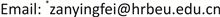Received: Feb. 12th, 2019; accepted: Feb. 23rd, 2019; published: Mar. 6th, 2019ABSTRACT

In this paper, the path planning of dynamic positioning ship is studied by numerical simulation. Firstly, the mathematical model of the ship is established. Then the tracking strategy is generated by setting the geometric position relation between the path generated by the path point and the ship, and the desired heading of the ship at the current moment is calculated. The control moment needed to control the ship to the desired heading is obtained by using the control algorithm. Finally, the longitudinal thrust required to control the ship to reach the desired velocity is calculated. At the same time, the motion response and propeller thrust change of the ship moving along the planned path under the action of waves are analyzed.

Keywords:Path Planning, Dynamic Position, Numerical Simulation, Motion Response

1中国船舶重工集团公司第七O三研究所，黑龙江 哈尔滨

2哈尔滨工程大学船舶工程学院，黑龙江 哈尔滨

3中国舰船研究院，北京Copyright © 2019 by author(s) and Hans Publishers Inc.1. 引言

2. 船舶时域运动学模型

$\left(M+{M}_{a}\right)\stackrel{˙}{v}+{\int }_{0}^{t}{K}_{ij}\left(t-\tau \right){v}_{j}\left(\tau \right)\text{d}\tau +g\left(\eta \right)={F}_{e}+{F}_{DP}+{F}_{l}$ (1)

3. 船舶路径规划模型

3.1. 动力定位系统

${F}_{DP}={K}_{p}\stackrel{˜}{\eta }+{K}_{i}{\int }_{0}^{t}\stackrel{˜}{\eta }\left(\tau \right)\text{d}\tau +{K}_{d}\stackrel{˜}{v}$ (2)

$J=\underset{i=1}{\overset{m}{\sum }}{W}_{i}\left({u}_{i}\right)+{s}^{T}Qs+{\left(\alpha -{\alpha }_{0}\right)}^{T}\Omega \left(\alpha -{\alpha }_{0}\right)$ (3)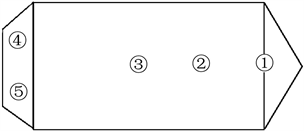Figure 1. Propeller distribution of DP ship

3.2. 路径规划算法研究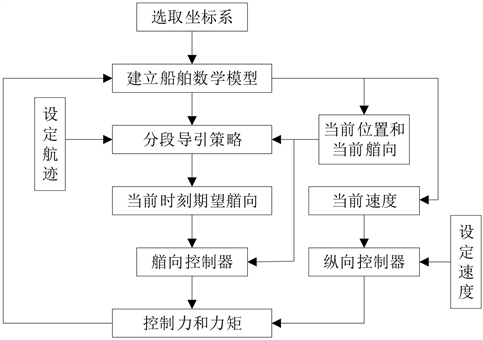Figure 2. Flow chart of Path planning algorithm

${\psi }_{d}={\mathrm{tan}}^{-1}\left(\frac{{y}_{los}-y}{{x}_{los}-x}\right)$ (4)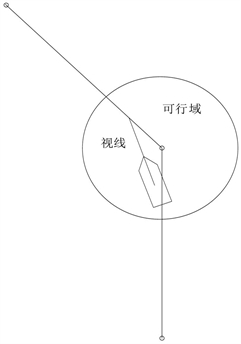Figure 3. Ship moment in circle of acceptance

4. 数值仿真与结果分析

4.1. 环境参数及路径点参数

4.2. 船舶路径规划运动分析

4.2.1 . 航迹分析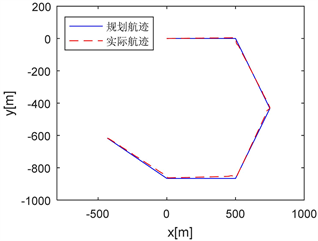Figure 4. Ship path planning track comparison chart

4.2.2 . 船舶运动响应分析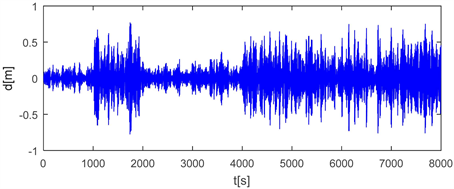Figure 5. Heave motion of ship curve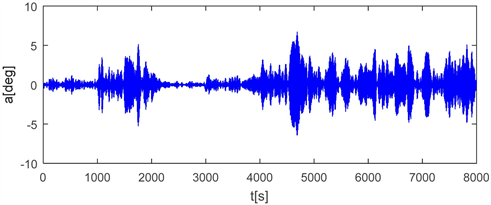Figure 6. Roll motion of ship curve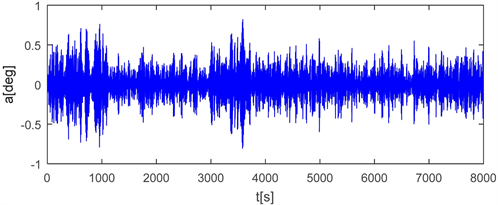Figure 7. Pitch motion of ship curve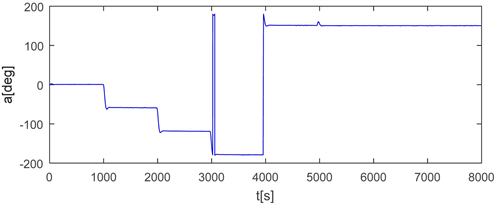Figure 8. Yaw motion of ship curve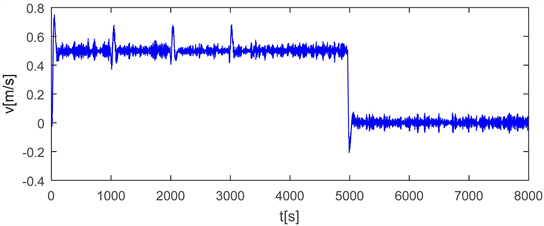Figure 9. Velocity of ship curve

4.2.3. 船舶推进器推力分析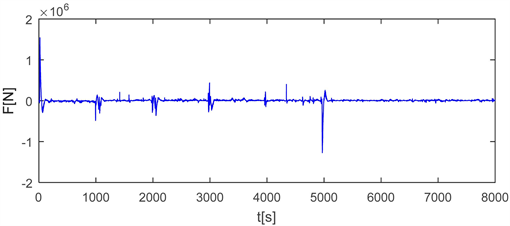Figure 10. x-thrust curve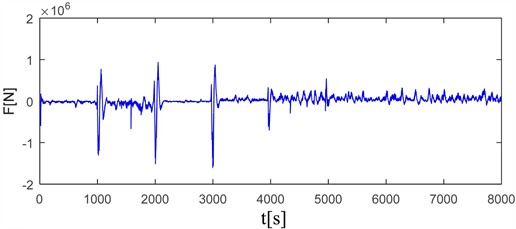Figure 11. y-thrust curve

5. 结论

Numerical Simulation of Path Planning in Ship[J]. 动力系统与控制, 2019, 08(02): 55-62. https://doi.org/10.12677/DSC.2019.82007

1. 1. 王宁. 世界海难事故现状分析及应对措施[J]. 世界海运, 2010, 33(7): 70-71.

2. 2. 郑恒, 白雪. 解秘“无人船”的前世今生[J]. 上海信息化, 2015(4): 24-27.

3. 3. 康与涛, 朱大奇, 陈伟炯. 船舶避碰路径规划研究综述[J]. 船海工程, 2013, 42(5): 141-145.

4. 4. Fossen, T.I. (2011) Handbook of Marine Craft Hydrodynamics and Motion Control. Wiley, Hoboken.
https://doi.org/10.1002/9781119994138

5. 5. 孙丽萍, 朱枭猛, 艾尚茂. 水下设备悬垂法安装动力定位耦合数值模拟[J]. 哈尔滨工程大学学报, 2017, 38(11): 1689-1695.

6. 6. 李严, 李素美, 刘苏粤. “海洋石油286”船三级动力定位系统配置分析[J]. 广东造船, 2017, 36(3): 33-36.

7. 7. MARINTEK (2013) SIMO-Theory Manual. Norwegian Marine Technology Research Insti-tute.

8. NOTES

*通讯作者。